# Excel中Sumprodcut函数用法大全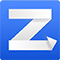Sumproduct函数的本职工作是返回两组数乘积的和，如：
=SUMPRODUCT(B2:B7,C2:C7)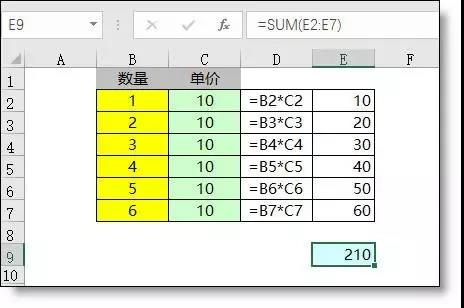01.插足单条件计数（抢Countif饭碗）

=SUMPRODUCT((A2:A5=B8)*1)=SUMPRODUCT((B3:B9="A")*C3:C9)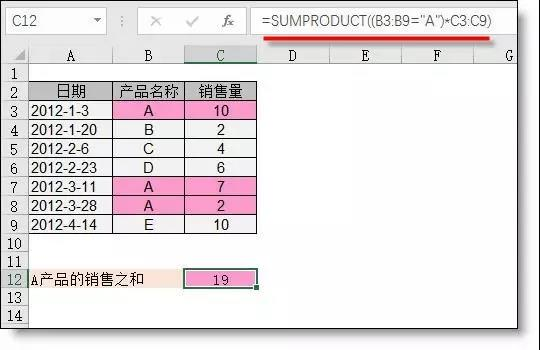03.插足多条件计数（抢Countifs饭碗）

=SUMPRODUCT((B3:B9="A")*(MONTH(A3:A9)=3))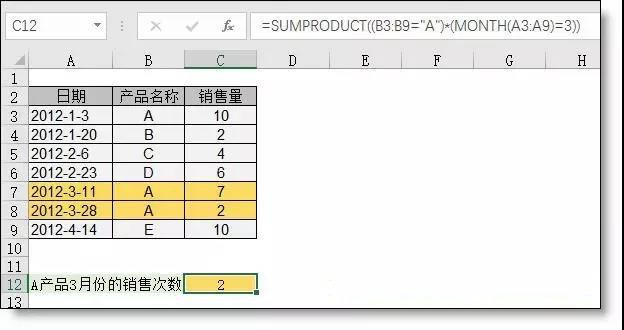04.插足多条件求和（抢Sumifs饭碗）

=SUMPRODUCT((B3:B9="A")*(MONTH(A3:A9)=3)*C3:C9)05.插足多条件查找（抢Lookup饭碗）

=SUMPRODUCT((A2:A5=A8)*(B2:B5=B8)*C2:C5)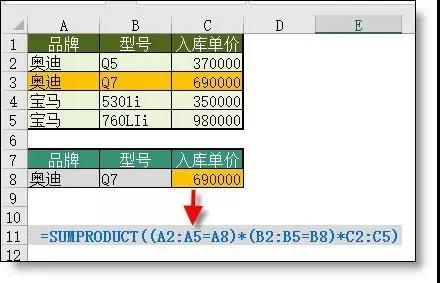06.插足数组求和（抢数组公式饭碗）

=SUMPRODUCT(1/COUNTIF(A2:A7,A2:A7))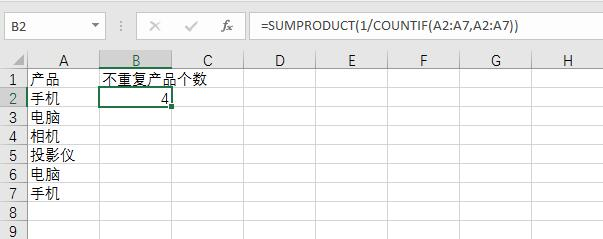=SUMPRODUCT(B2:B10*1)